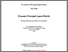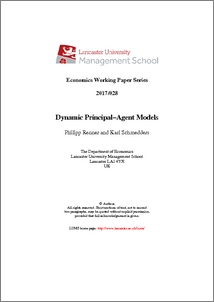# Dynamic Principal–Agent Models

Renner, Philipp and Schmedders, Karl (2017) Dynamic Principal–Agent Models. Working Paper. Lancaster University, Department of Economics, Lancaster.Preview
PDF (LancasterWP2017_028)
LancasterWP2017_028.pdf

## Abstract

This paper contributes to the theoretical and numerical analysis of discrete time dynamic principal-agent problems with continuous choice sets. We first provide a new and simplified proof for the recursive reformulation of the sequential dynamic principal-agent relationship. Next we prove the existence of a unique solution for the principal's value function, which solves the dynamic programming problem in the recursive formulation. By showing that the Bellman operator is a contraction mapping, we also obtain a convergence result for the value function iteration. To compute a solution for the problem, we have to solve a collection of static principal{agent problems at each iteration. Under the assumption that the agent's expected utility is a rational function of his action, we can transform the bi-level optimization problem into a standard nonlinear program. The final results of our solution method are numerical approximations of the policy and value functions for the dynamic principal-agent model. We illustrate our solution method by solving variations of two prominent social planning models from the economics literature.

Item Type:
Monograph (Working Paper)
Subjects:
Departments:
ID Code:
88600
Deposited By:
Deposited On:
07 Nov 2017 16:28
Refereed?:
No
Published?:
Published